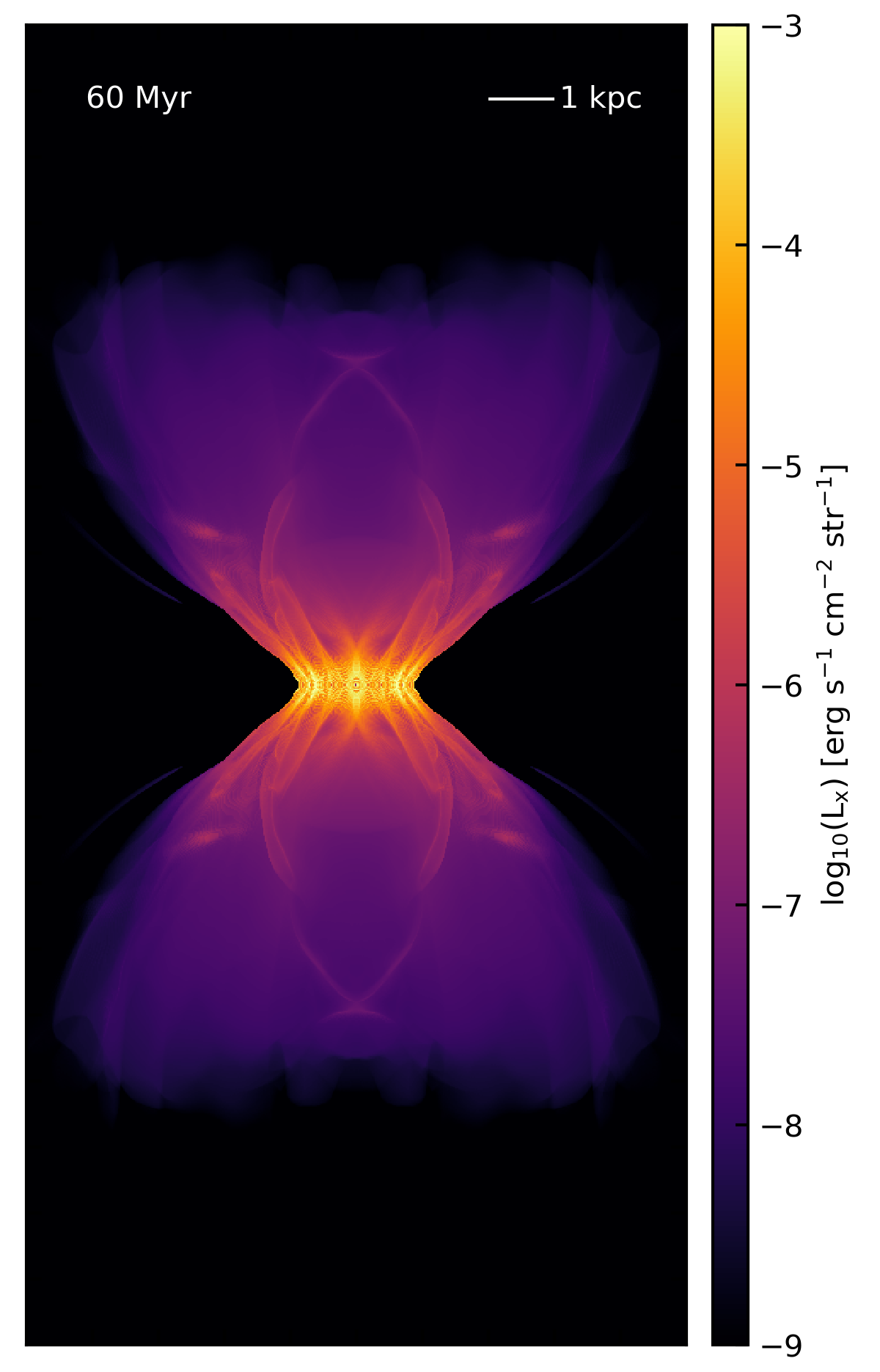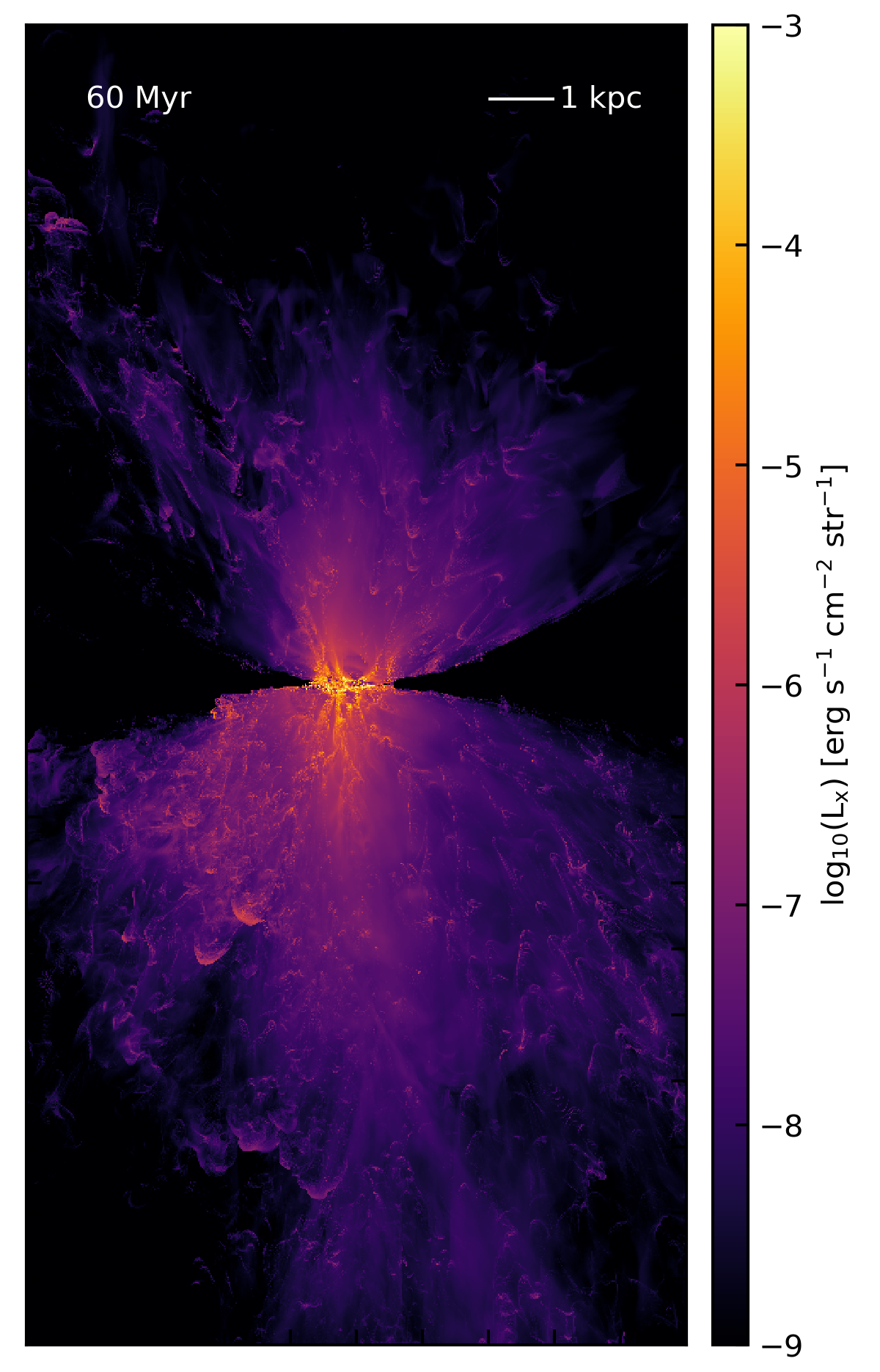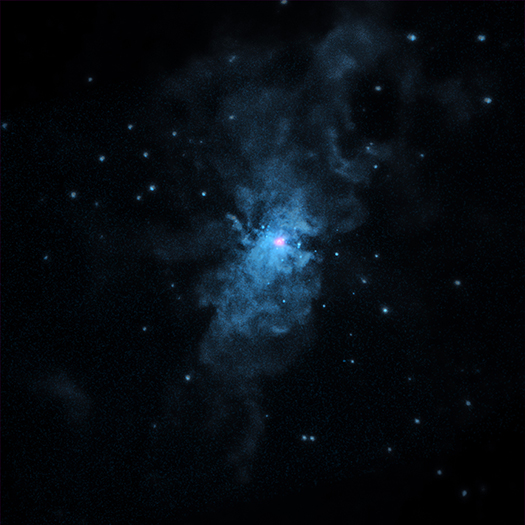# X-ray Emission

## January 29, 2018

In an attempt to connect the outflow simulations I’ve been doing to observations, I’ve decided to try to model the x-ray emission from the hot plasma and compare it to what’s seen in M82. I’ll start with a simple model for the diffuse soft x-ray emission. Briefly, for a given cell in the simulation, I’ve got a density $\rho$, that I can convert to a number density, $n$, by assuming that the gas is ionized, so $n = \rho / \mu m_p$, where $\mu = 0.6$ and $m_p$ is the mass of a proton. Given this number density, I can also calculate a volume-averaged temperature for the gas in the cell, $T = e (\gamma - 1.0) / n k_B$, where $e$ is the internal energy density (tracked in the dual-energy version of Cholla), $\gamma$ is the adiabatic index (assumed to be 5/3), and $k_B$ is the Boltzmann constant. I can then calculate the total radiative luminosity from the cell via a cooling function $\Lambda$.

I’ll start with the analytic cooling function that was used for these simulations, which is a fit to a CIE curve made with Cloudy assuming solar abundances. I can then create a surface brightness map for the soft X-rays by integrating $n^2 \Lambda$ along the line of sight for a given projection in my simulation. Only cells with temperatures in the soft X-ray band are included in the integration. I convert from temperature to energy by assuming $E = \frac{3}{2} k_B T$, as appropriate for a monatomic gas. This results in a brightness in $\mathrm{erg}\,\mathrm{s}^{-1}\,\mathrm{cm}^{-2}$. Dividing by $4\pi$ steradian gives a surface brightness. The resulting surfaxe brightness map for the $512\times 512 \times 1024$ adiabatic simulation at 60 Myr with central injection feedback looks like this:Clearly, the soft x-rays are highly collimated in this simulation. The additional features that show up in the x-ray map are from interacting shocks within the simulation volume. At this resolution, the large-scale evolution of the simulation is not yet converged. At higher resolutions, the disk-outflow interface region becomes highly turbulent, leading to more filamentary structures (figure coming soon). Interestingly, the soft X-ray luminosity integrated over the entire simulation volume is $L_x = 2.9\times10^{40}$, which is suprisingly close to the total oberserved luminosity. For example, Strickland et al. (2004) calculate an observed diffuse soft X-ray luminosity for M82 of $L_x = 4.3\times10^{40}$ (see their Table 9).

Here’s the surface brightness map for the 512-resolution clustered feedback simulation (which includes cooling), so is actually self-consistent:Here we see less collimation. There is also significant assymetry, with the soft X-rays extending farther in radius in the lower half of the simulation volume. The total X-ray luminosity for this simulation is lower, $L_x = 4.5\times10^{39}$, though this is still within an order of magnitude of the observations. (Also, as noted above, these values are not converged - see the next post for results of the higher resolution simulations.) The overall morphology of the X-ray emission compares favorably to the emission seen in M82. For example, here is an image of M82 in soft X-rays made with Chandra:In the next post I’ll explore the radial profiles of the surface brightness for the two models.